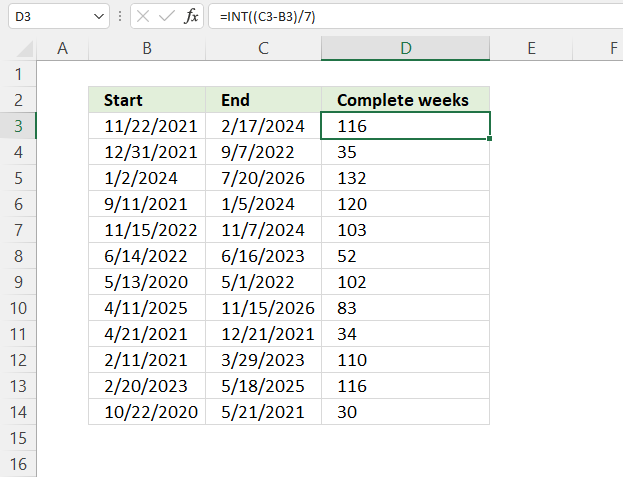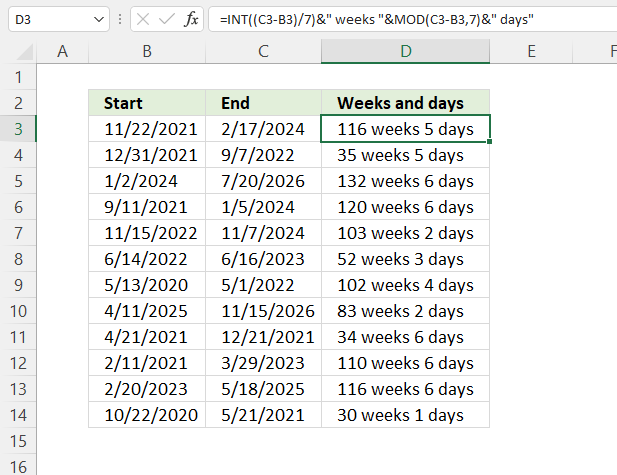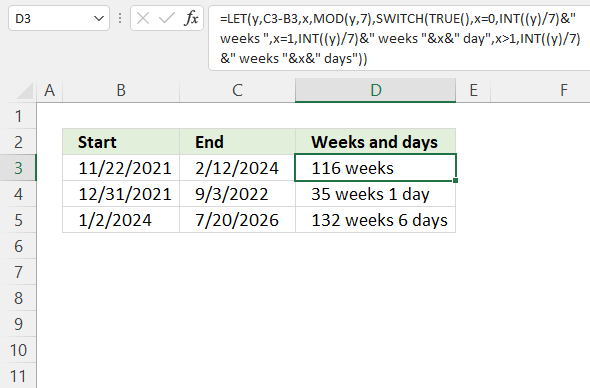Author: Oscar Cronquist Article last updated on April 25, 2023This article demonstrates Excel formulas that calculate complete weeks between two given dates and weeks and days between two given dates.

## 1. Calculate the number of weeks between given datesThe image above demonstrates a formula that calculates the number of complete weeks between two dates. Cell range B3:B14 contains the start dates and cell range C3:C14 contains the end dates.

Formula in cell D3:

=ROUNDDOWN((C3-B3)/7)

Copy cell D3 and paste to cells below as far as needed.

### 1.1 Explaining formula in cell D3

This formula works fine if the start is later than the end date, however, you get a minus sign before the number.

If you want to remove the minus sign simply use the ABS function to remove it, the formula then becomes:

=ABS(ROUNDDOWN((C3-B3)/7))

#### Step 1 - Subtract dates

C3-B3

becomes

35067 - 35685 equals 618 days.

#### Step 2 - Divide with 7

There are seven days in a week so we need to divide the result with 7.

(C3-B3)/7

becomes

618/7 equals 88.28571429.

#### Step 3 - Round the number down

The ROUNDDOWN function rounds the number down.
ROUNDDOWN(numbernum_digits)

ROUNDDOWN((C3-B3)/7)

becomes

ROUNDDOWN(88.28571429) and returns 88.

## 2. Calculate the number of weeks and days between given datesThis formula returns the total number of weeks and days between a given start and end date.

Formula in cell D3:

=INT((C3-B3)/7)&" weeks "&MOD(C3-B3,7)&" days"

### 2.1 Explaining formula

#### Step 1 - Calculate days between dates in cells C3 and B3

The minus sign lets you subtract numbers in an Excel formula.

C3-B3

becomes

45339-44522

and returns 817.

#### Step 2 - Calculate weeks

The division character lets you divide numbers in an Excel formula. The parentheses let you control the order of operation, we want to subtract before we divide.

(C3-B3)/7

becomes

817/7

and returns approx. 116.71

#### Step 3 - Remove decimals

The INT function removes the decimal part from positive numbers and returns the whole number (integer) except negative values are rounded down to the nearest integer.
INT(number)

INT((C3-B3)/7)

becomes

INT(116.71)

and returns 116

#### Step 4 - Concatenate number and string

The ampersand character lets you concatenate values in an Excel formula. Use double quotes with text values to avoid a formula #NAME error.

INT((C3-B3)/7)&" weeks "

becomes

116&" weeks"

and returns 116 weeks.

#### Step 5 - Calculate the remainder

The MOD function returns the remainder after a number is divided by a divisor.
MOD(numberdivisor)

MOD(C3-B3, 7)

becomes

MOD(817, 7)

and returns 5.

#### Step 6 - Concatenate numbers and text values

The ampersand character concatenates values in an Excel formula.

INT((C3-B3)/7)&" weeks "&MOD(C3-B3,7)&" days"

becomes

116&" weeks "&5&" days"

and returns 116 weeks 5 days.

## 3. Calculate the number of weeks and days between given dates - dynamic text valuesThis formula works only in Excel 365, it calculates weeks and days between a given start and end date. It is also dynamic meaning if the result is a whole week the number of days is left out from the output.

Excel 365 formula in cell D3:

=LET(y,C3-B3,x,MOD(y,7),z,INT((y)/7),q," weeks ",SWITCH(TRUE(),x=0,z&q,x=1,z&q&x&" day",x>1,z&q&x&" days"))

### Explaining formula

#### Step 1 - First argument expression

The SWITCH function returns a given value determined by an expression and a list of values. The SWITCH function is made for exact matches, however, there is a workaround to use larger than and smaller than characters.

If any of the value arguments returns a value equal to the expression argument the corresponding result argument is returned.

SWITCH(expressionvalue1result1, [default or value2result2],…[default or value3result3])

TRUE and FALSE are boolean values, they are often the result of a logical test. I am going to use TRUE in this expression argument.

#### Step 2 - Second argument value1

The following formula calculates the remaining days after we subtract two Excel dates and then divide by seven, there are seven days in one week.

The MOD function returns the remainder after a number is divided by a divisor.
MOD(numberdivisor)

MOD(C3-B3,7)=0

becomes

MOD(44522-45334, 7)=0

becomes

MOD(812, 7)=0

becomes

0=0

and returns TRUE. This value matches the expression argument, the formula will now return the result argument.

#### Step 3 - Third argument result1

The INT function removes the decimal part from positive numbers and returns the whole number (integer) except negative values are rounded down to the nearest integer.
INT(number)

INT((C3-B3)/7)&" weeks "

becomes

INT((812)/7)&" weeks "

becomes

INT(116)&" weeks "

becomes

116&" weeks "

and returns "116 weeks" in cell D3.

There are two more value arguments:
MOD(C3-B3,7)=1 adds day to the result. The remainder is one.
MOD(C3-B3,7)>1 adds days to the result. The remainder is more than one.

#### Step 4 - Shorten the formula

The LET function allows you to name intermediate calculation results which can shorten formulas considerably and improve performance.
LET(name1name_value1calculation_or_name2, [name_value2calculation_or_name3...])

SWITCH(TRUE(),MOD(C3-B3,7)=0,INT((C3-B3)/7)&" weeks ",MOD(C3-B3,7)=1,INT((C3-B3)/7)&" weeks "&MOD(C3-B3,7)&" day",MOD(C3-B3,7)>1,INT((C3-B3)/7)&" weeks "&MOD(C3-B3,7)&" days")

I have named intermediate calculations if they are repeated in the formula, this creates a shorter formula.

y - C3-B3
x - MOD(y,7)
z - INT((y)/7)
q - " weeks "

LET(y,C3-B3,x,MOD(y,7),z,INT((y)/7),q," weeks ",SWITCH(TRUE(),x=0,z&q,x=1,z&q&x&" day",x>1,z&q&x&" days"))